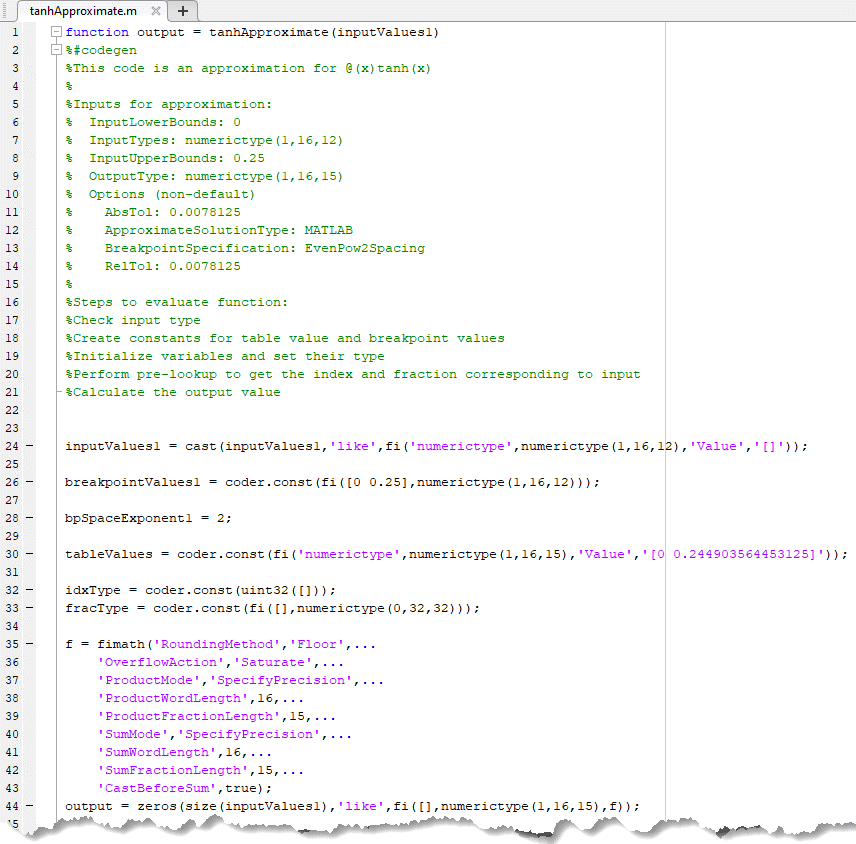# Generate an Optimized Lookup Table as a MATLAB Function Programmatically

This example shows how to generate an optimized lookup table as a MATLAB® function to approximate hyperbolic tangent. The MATLAB function lookup table approximation can then be used to replace the hyperbolic tangent function and generate C code.

Use the `FunctionApproximation.Options` object to specify a MATLAB function as the solution type. Use the default values for accuracy and word length constraints.

```options = FunctionApproximation.Options(); options.ApproximateSolutionType = 'MATLAB';```

Specify the function to approximate and the input ranges and data types in the `FunctionApproximation.Problem` object.

```functionToApproximate = 'tanh'; problem = FunctionApproximation.Problem(functionToApproximate, 'Options', options); problem.InputLowerBounds = 0; problem.InputUpperBounds = 0.25;```

Use the `solve` method to solve the optimization problem and create a lookup table solution.

`solution = solve(problem)`
```Searching for fixed-point solutions. | ID | Memory (bits) | Feasible | Table Size | Breakpoints WLs | TableData WL | BreakpointSpecification | Error(Max,Current) | | 0 | 64 | 1 | 2 | 16 | 16 | EvenSpacing | 7.812500e-03, 1.962196e-03 | | 1 | 64 | 1 | 2 | 16 | 16 | EvenPow2Spacing | 7.812500e-03, 1.962196e-03 | Best Solution | ID | Memory (bits) | Feasible | Table Size | Breakpoints WLs | TableData WL | BreakpointSpecification | Error(Max,Current) | | 1 | 64 | 1 | 2 | 16 | 16 | EvenPow2Spacing | 7.812500e-03, 1.962196e-03 | ```
```solution = 1x1 FunctionApproximation.LUTSolution with properties: ID: 1 Feasible: "true" ```

To obtain the generated lookup table as a MATLAB function, use the `approximate` method. Use optional name-value arguments to specify the name and path for the lookup table function.

```filename = 'tanhApproximate'; filepath = cd; approximate(solution,'Name',filename,'Path',filepath);```If you have MATLAB Coder™ installed, you can use the `codegen` command to generate C code from the approximate lookup table function.

```inputArgs = linspace(1,10,10); codegen tanhApproximate.m -args {inputArgs}```
```Code generation successful. ```